﻿ ANFIS Based Identification and Control of Distillation Process

### ANFIS Based Identification and Control of Distillation Process

M. Shahbazian, H. Jazayerirad, M. EbnaliOPEN ACCESSPEER-REVIEWED

## ANFIS Based Identification and Control of Distillation Process

M. Shahbazian1, H. Jazayerirad1, M. Ebnali1,1Department of Instrumentation and Automation Engineering, Ahwaz Faculty of Petroleum Engineering, Ahwaz, Iran

### Abstract

Typical production objectives in distillation process require the delivery of products whose compositions meets certain specifications. The distillation control system, therefore, must hold products composition as near the set points as possible in the faces of upsets. Since product quality cannot be measured easily and economically online, the control of product quality is often achieved by maintaining a suitable tray temperature near its set point. Tray temperature control method, however, is not a proper option for a multi-component distillation column, because the tray temperature does not correspond exactly to the product composition. To overcome this problem, secondary measurements can be used to infer the product quality and adjust the values of the manipulated variables. In this paper we have used a novel adaptive network fuzzy inference system (ANFIS) based inferential control approach for distillation process. ANFIS is used for identifying the distillation process and building two composition estimators to estimate the compositions of the bottom and sidestream products. The developed estimators are tested and results show that the predictions made by ANFIS structure are in good agreement with the results of simulation by ASPEN HYSYS process simulation package. In addition inferential control by implementation of ANFIS based online composition estimators is more superior to traditional tray temperature control method because of less integral time absolute error.

### At a glance: Figures

123
Prev Next

• Shahbazian, M., H. Jazayerirad, and M. Ebnali. "ANFIS Based Identification and Control of Distillation Process." Journal of Automation and Control 2.2 (2014): 49-56.
• Shahbazian, M. , Jazayerirad, H. , & Ebnali, M. (2014). ANFIS Based Identification and Control of Distillation Process. Journal of Automation and Control, 2(2), 49-56.
• Shahbazian, M., H. Jazayerirad, and M. Ebnali. "ANFIS Based Identification and Control of Distillation Process." Journal of Automation and Control 2, no. 2 (2014): 49-56.

 Import into BibTeX Import into EndNote Import into RefMan Import into RefWorks

### 1. Introduction

Distillation of multi-component mixtures is one of the most common separation operations in the chemical industry. The distillation control system must hold product composition as near the set point as possible in the faces of upsets. The control is difficult because the product quality cannot be measured easily and economically online. This is because the instrumentation is either very expensive and / or measurement lags and sampling delays make impossible to design an effective control system.

A solution to this problem is the use of tray temperature control method. The temperature control is based on the assumption that the product composition can satisfy its specification when an appropriate tray temperature is kept constant at its set point. If tray temperatures are to be used, the issue is selecting the best tray or trays on which temperature is held constant. This problem has been discussed in the distillation literature for over a half century. The pioneering books by Buckley , Rademaker et al.  and Shinskey  present a number of alternative procedures for making this selection. Hundreds of papers have appeared that use temperature control with each author using one particular method for control tray selection. A recent discussion of one approach to this problem is given in Marlin .

Tray temperature control method is a proper option for binary distillation columns at constant pressure, but in the case where feed composition or feed flow rate changes in a multi-component distillation tower, it is quiet difficult to keep product quality at its set point by using temperature control, because the tray temperature does not correspond exactly to the product composition. In addition pressure changes also cause temperature variation. To solve this problem, secondary measurements in conjunction with a mathematical/empirical model of process can be used to estimate the product quality.

The distillation Process can be modeled using input-output data from experimental tests. Regression and neural network modeling technique are commonly used for this purpose. Morris et al.  examined the contribution that various network methodologies can make to the process modeling and control toolbox. Feed forward networks with sigmoidal activation functions, radial bases function networks and auto associative networks were reviewed and studied using data from industrial processes. The concept of dynamic networks was introduced with an example of nonlinear predictive control.

MacMurray et al.  described the modeling of packed distillation column with artificial neural network (ANN) and provide an example of complex modeling. The change in the sign of the gain was observed under various operating conditions.

Kung et al.  proposed algebraic projection analysis and provide an analytical solution for optimal hidden units size and learning rate of the back propagation neural networks. Baratti et al  used neural networks to control distillation column.

Singh et al.  by using artificial neural network estimated composition of distillate in distillation column. The structure of neural network has been made by input and output data, which inputs are temperatures and outputs are composition of distillate. Artificial neural networks were applied to predict and estimate vapor–liquid equilibrium data for ternary systems saturated with salt by Nguyen et al. .

Tamura et al.  have discussed the capabilities of a neural network with a finite number of hidden units and shown with the support of mathematical proof that a four layered feed forward network is superior to three layered feed forward network in terms of the number of parameters needed for the training data.

Murata et al.  have investigated the problem of determining the optimal number of parameters in neural network from statistical point of view. The proposed new information criterion therein measures the relative merits of two models having the same structure but different number of parameters and concludes whether more number of neurons should be added to the network or not.

Kano et al.  presented a control scheme to control the product composition in a multi-component distillation column. The distillate and bottom compositions are estimated from online measured process variables. The inferential models for estimation product compositions are constructed using dynamic partial least squares (PLS) regression, on the base of simulated time series data. From the detailed dynamic simulation results, it is found that the cascade control system based on a proposed dynamic (PLS) model works much better than the usual tray temperature control system.

Kano et al.  proposed a new inferential control scheme termed as “Predictive Inferential Control”. In predictive inferential control system, future compositions predicted from online measured process variables are controlled instead of the estimates of current compositions. The key concept is to realize the feedback control with a feed forward effect by the use of inherent nature of a distillation column.

Sbarbaro et al  presented the traditional approach to include multi-dimensional information into conventional control systems and proposed a new structure based on pattern recognition. The artificial neural networks and finite state machines as a frame work for designing the control system is used.

Taking advantage of technique developed by Kolmogorov, Kurkova  provided a direct proof of the universal approximation capabilities of perceptron type network with two hidden layers.

Lippmann  demonstrated the computational power of different neural net models and the effectiveness of simple error correction training procedures. Single and multilayer perceptrons, which can be used for pattern classification, are described as well as Kohonen’s feature map algorithm, which can be used for clustering or as a vector quantizer.

To our knowledge, there is no ANFIS based inferential control approach for distillation process. The main aim of this paper is to present a novel identification and control study concerning an industrial distillation column using adaptive network fuzzy inference systems. ANFIS structure is used for building two nonlinear models of the distillation column. The investigated column operates the separation of benzene from the outlet stream of a reforming unit. The benzene concentration in both the bottom and sidestream has to be controlled.

The research work was carried out in the following steps: (i) steady state simulation of the process; (ii) dynamic simulation of process; (iii) identification of process; (iv) inferential control of process based on empirical model of the system and (v) comparison between the proposed inferential control and the traditional tray temperature control strategy.

### 2. Methods

2.1. Steady State Simulation of the Process

A schematic representation of the examined column is given in Figure 1. The column has 21 valve trays: feed enters at tray 4 and bottom, sidestream and top products are removed at trays 1, 16, 21 respectively. Composition of the feed stream is illustrated in table 1. The column was simulated by means of ASPEN HYSYS process simulation package and steady state operating conditions of column are reported in table 2.

#### Table 1. Composition of column feed

The column feed stream is the outlet stream from the reforming unit. The purpose of this column is to reduce the benzene concentration in the reformed bottom product gasoline, which is sent to the blending unit.

#### Table 2. Steady state operating conditions of the column

2.2. Dynamic Simulation of the Process

The dynamic behavior of the column was simulated by ASPEN HYSYS DYNAMIC simulation package. Tray liquid hydraulic was predicted by Glitsch procedure and the equilibrium constants were calculated by SRK relation. The simulation model takes into account the distillation tower as well as all the auxiliary apparatus (reboiler, condenser, reflux drum, measuring devices and control elements).

As illustrated in Figure 2, for design of distillation column control system, it is proposed that the sidestream flow rate is manipulated to control the tray 17 temperature in order to keep the benzene concentration in the side stream constant, the reflux drum liquid level is controlled by manipulating the distillate flow rate, reboiler liquid level is controlled by manipulating bottom product flow rate and finally tray 2 temperature is controlled by manipulating the reboiler duty in order to keep the bottom product benzene concentration constant.

2.3. Process Identification
2.3.1. Input signal design and data collection

Simulated data for building inferential estimators are obtained under the following conditions. A connection is established between MATLAB and HYSYS software. The pseudo random binary signals of bounded and varying amplitude (within ±5% of the steady state values) are introduced by MATLAB as feed temperature and total feed flow rate changes during dynamic simulation of the column by HYSYS simulator. For realizing slow composition changes, each signal is filtered by a first order lag model. Total simulation time is 8 hours and process data are sampled every 10 seconds by MATLAB.

Simulated data for validating inferential models are obtained under almost the same conditions as described above. Total simulation time is 4 hours.

If the input-output data described above are obtained when the temperature controllers are in the automatic mode, the data do not include the operational conditions when the controlled temperatures undergo large changes. However, when inferential composition control is applied instead of temperature control, the trays temperature fluctuates greatly. Thus, when an inferential model is used for composition control, the accuracy of estimation may deteriorate due to large changes of tray temperature. In order to improve the accuracy, the inferential model must be built using appropriate data, which include large fluctuation of the temperature. For this purpose, the proportional gain of bottom temperature controller is changed between ±50% of base controller gain in the simulation in order to change reboiler duty. Figure 3 represents the input signals applied to the process for collecting identification data.

2.3.2. Data Preprocessing

Collected data have different magnitudes depending on the units adopted. This can cause larger magnitude variables to be dominant over smaller ones during the training process. Data scaling is therefore needed. Min- max normalization method is one of the common scaling methods. Min-max normalization is given by:(1)

Where

a is unscaled variable;

a’ is scaled variable

mina is minimum value of unscaled variable

maxa is maximum value of unscaled variable

mina is minimum value of scaled variable

maxa is maximum value of scaled variable

In this research, collected data are scaled to [0,1] interval. Since collected data are the results of simulation and have no inconsistent data, so other preprocessing operation such as outlier detection, missing value replacement and data de-noising are not required.

2.3.3. Model Structure Selection

One modification of a neural network structure is to replace some or all components of neuron by fuzzy logic operations. Conventional neural networks are used to approximate functions from numerical input-output data. Fuzzy-neural networks are a more general computational structure with which function approximation can be extended to linguistic data.

To illustrate the use of neural networks for fuzzy inference, consider a fuzzy rule base consisting of only two Sugeno-Takagi rules:(2)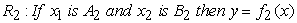(3)

Where Ai and Bi are fuzzy sets and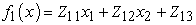(4)(5)

When numerical input x=(x1,x2) is presented, the inference mechanism will produce the numerical output(6)

A fuzzy neural network for implementing the above formula is shown in Figure 4. The observed input x=(x1,x2) is presented to layer 1 by input layer 0. The output of layer 1 is:(7)

Layer 2 consists of fuzzy neurons with an aggregation operator being some T-norm. If product T-norm is used the output of layer 2 is: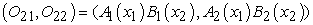(8)

Layer 3 is normalizer. The output of layer 3 is: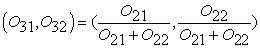(9)

The fuzzy neurons in layer 4 output the values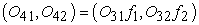(10)

Finally the output layer calculates the estimated output by summing: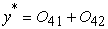(11)

Of course, this neural network type for representing the inference procedure for a rule base of two rules can be extended in an obvious way to an arbitrary number of rules.

In the ANFIS structure, the parameters of the premise and consequence play the role of weights in neural network systems. The ANFIS learning algorithm consists of adjusting the mentioned parameters from sample data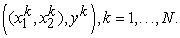2.3.4. Validation of Model

The estimated model can be evaluated on the basis of mean squared error of prediction (MSEP), which is calculated by applying the models to the validation data.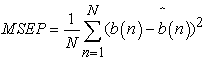(12)

Where b is a measurement of the product composition,is its estimate and N is the number of measurements.

### 3. Results and Discussion

3.1. Inferential Models

The output variables to be estimated are the benzene concentration in bottom and sidestream products. The inputs to the inferential estimators are various combinations of 21 tray, condenser and reboiler temperatures and feed, distillate, bottom and sidestream flow rates. Table 3 illustrates the properties of fuzzy inference systems and MSEP of validation data for the following combinations of input variables to the inference systems (cases A1 to A10 for estimation of bottom product benzene concentration and cases B1 to B10 for estimation of sidestream benzene concentration).#### Table 3. Fuzzy inference system properties and MSEP for some combination of inputs to ANFIS structure

Examination of various combinations of inputs show that combinations recommended in cases A10 and B10 are the most efficient for estimation of Benzene concentration in the bottom product and sidestream, respectively, based on MSEP. Figure 5 and Figure 6 visualize the estimated values versus the actual ones for both training (samples number from1 to 2880) and validation data (samples number from 2881 to 4320) of mentioned cases.

3.2. Control Strategy and Results

On the basis of the control analysis reported in section 2.2, the inferential control strategy of the column shown in Figure 7 was adopted. A cascade control scheme has been assumed for each control variable, with benzene concentration on the primary (master) loop and tray temperature on the secondary (slave) one. The efficiency of the control strategy was examined on the basis of the responses of the process to step change of the benzene composition of the feed stream (±10%), feed temperature (±5%) and feed total mass flow rate (±5%). The evaluation of control efficiency was done by calculating the integral time absolute error (ITAE) (see table 4) over a horizon of 3 hours after the implementation of disturbance.

The response of the system to step change in feed stream benzene concentration (+10%), when the benzene concentration of only one product stream is controlled by the cascade inferential scheme or the benzene concentration of two product streams are controlled by the cascade inferential scheme (full inferential) and also keeping constant the relevant tray temperature were compared in Figure 8 and Figure 9. It can be observed that:

The traditional tray temperature control scheme lead to the significant offset for both the bottom and sidestream.

The response of full inferential scheme and single variable inferential scheme are very close to each other.

Figure 8. Comparison between the performance of the traditional tray temperature, full inferential and single variable inferential bottom product controller in response to step change in feed stream benzene concentration
Figure 9. Comparison between the performance of the traditional tray temperature, full inferential and single variable sidestream composition controller in response to step change in feed stream benzene concentration (+10%)

#### Table 4. ITAE for benzene concentration for different control schemes and different disturbances

At the end of transient state a small offset of sidestream benzene concentration can be observed when both the single variable and full inferential strategies are applied. This is due to the inability of sidestream composition estimator to estimate as accurate as bottom composition estimator (see Figure 5 and Figure 6). The value of offset is greater when full inferential scheme is used. This is the consequence of interaction between the individual control loops (coupling problem).

### 4. Conclusion

Distillation column is one of the equipments is used in chemical industry. Products of this column must have specific composition concentration. The devices for measuring the product composition are costly and have high delay time. Inferential control by using of secondary measurements in conjunction with an empirical model of process can be the replacement of direct composition control method.

In this research, a column with 21 trays, condenser and reboiler is studied. 4320 data is generated by implementation of proper disturbances to the process. 2880 data is used for training of ANFIS based composition estimators and 1440 data is used for validation of the models. The MSEP criterion is used to identify the optimal combination of input variables to inference system.

It is concluded that the use of ANFIS based composition estimators leads to the predictions that are in good agreement with the results of simulation by ASPEN HYSYS process simulation package, also inferential control of distillation column by implementation of ANFIS based online composition estimators is more superior to traditional tray temperature control method because of less integral time absolute error.

### Nomenclature

B: Bottom Stream

BF: Bottom Stream Flow Rate (Kg/hr)

BZ: Benzene

CC: Composition Controller

D: Distillate Stream

F: Feed Stream

FF: Feed Stream Flow Rate (Kg/hr)

IR: Internal Reflux Stream

LC: Level Controller

N: Number Of Samples

Q: Reboiler Duty (Kj/hr)

R: External Reflux Stream

RDT: Reflux Drum Temperature (C)

RebT: Reboiler Temperature (C)

SS: Side Stream

SSF: Sidestream Flow Rate (Kg/hr)

SP: Set Point

TC: Temperature Controller

Ti: Tray i Temperature (C)

Wt: Weight (Kg)

x: Linguistic Variable

X: Concentration In Weight Percent

Z: Constant coefficient

### References

  Baratti,R., Corti, S. and Servida, A., “A Feed forward Control Strategy for Distillation Columns,” Artificial Intelligent Engineering, 11. 405-412.1997.In article  Bettoni, A., Bravi, M. and Chianese, A. “Inferential Control of Sidestream Distillation Columns,” Computer and Chemical Engineering, 23. 1737-1744. 2000.In article CrossRef  Buckley, P.S, Techniques of Process Control, Wiley, 1964.In article  Kano, M., Showchaiya, N., Hasebe, S. and Hashimoto, I., “Inferential Control System of Distillation Compositions Using Dynamic Partial Least Square Regression,” Journal of Process Control, 10. 157-166. 2000.In article CrossRef  Kano, M., Showchaiya, N., Hasebe, S. and Hashimoto, I., “Inferential Control of Distillation Compositions: Selection of Model and Control Configuration,” Control Engineering Practice, 11. 927-933. 2003.In article CrossRef  Kung, S.Y and Hwang, J.N, An Algebraic Projection Analysis for Optimal Hidden Units Size and Learning Rates in Back Propagation Learning, Princeton University, Department of Electrical Engineering, Princeton, NJ 08544.In article  Kurkova, V., “Kolmogorov’s Theorem and Multi Layer Neural Networks,” Neural Networks, 5. 501-506. 1992.In article CrossRef  Lippmann, R.P, Neural Nets for Computing, Lincola Laboratory, M.I.T, Lexington, MA 02173, U.S.A.In article  MacMurray, J.C. and Himmelblau, D.M., “Modeling and Control of a Packed Distillation Column Using Artificial Neural Networks,” Computer and Chemical Engineering, 19.1088. 1995In article  Marlin, T.E, Process Control: Designing Processes and Control Systems for Dynamic Performance, McGraw-Hill, 2000.In article  Morris, A.J., Montague, G. A. and Willis, M.J., “Artificial Neural Networks: Studies in Process Modeling and Control,” Transaction of Institute of Chemical Engineering, 72 (A). 3-19. 1994.In article  Murata, N., Yoshizawa, S. and Amari, S., “Network Information Criterion Determining the Number of Hidden Units for an Artificial Neural Network Model,” IEEE Transaction of Neural Networks, 5. 865- 872.1994.In article  Nguyen, V.D., Tan, R.R., Brondial, Y. and Fuchino, T., “Prediction of Vapor-Liquid Equilibrium Data for Ternary Systems Using Artificial Neural Networks,” Fluid Phase Equilibra, 254. 188-197. 2007.In article CrossRef  Rademaker, O., Rijnsdorp, J.E. and Maarleveld, A., Dynamics and Control of Continuous Distillation Units, Elsevier, 1975.In article  Sbarbaro, D., Espinoza, P. and Araneda, J., “A Pattern Based Strategy for Using Multidimensional Sensors in Process Control,” Computer and Chemical Engineering, 27. 1943. 2003.In article  Shinskey, F.G, Distillation Control, McGraw-Hill, 1977.In article  Singh, V., Gupta, I. and Gupta, H.O. “ANN Based Estimator for Distillation Inferential Control,” Chemical Engineering Process, 44. 785-795. 2005.In article CrossRef  Tamura, S., Tateishi, M., “Capabilities of a Four Layered Feed Forward Neural Network: Four Layers Versus Three,” IEEE Transaction of Neural Networks, 8. 251-255.1997.In article